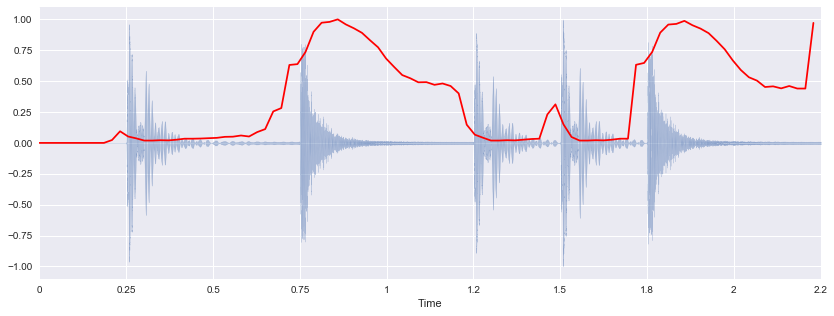In :
%matplotlib inline
import seaborn
import numpy, scipy, matplotlib.pyplot as plt, IPython.display as ipd, sklearn
import librosa, librosa.display
plt.rcParams['figure.figsize'] = (14, 5)


# Spectral FeaturesÂ¶

For classification, we're going to be using new features in our arsenal: spectral moments (centroid, bandwidth, skewness, kurtosis) and other spectral statistics.

Moments is a term used in physics and statistics. There are raw moments and central moments.

You are probably already familiar with two examples of moments: mean and variance. The first raw moment is known as the mean. The second central moment is known as the variance.

## Spectral CentroidÂ¶

In :
x, sr = librosa.load('audio/simple_loop.wav')
ipd.Audio(x, rate=sr)

Out:

The spectral centroid (Wikipedia) indicates at which frequency the energy of a spectrum is centered upon. This is like a weighted mean:

$$f_c = \frac{\sum_k S(k) f(k)}{\sum_k S(k)}$$

where $S(k)$ is the spectral magnitude at frequency bin $k$, $f(k)$ is the frequency at bin $k$.

librosa.feature.spectral_centroid computes the spectral centroid for each frame in a signal:

In :
spectral_centroids = librosa.feature.spectral_centroid(x, sr=sr)
spectral_centroids.shape

Out:
(97,)

Compute the time variable for visualization:

In :
frames = range(len(spectral_centroids))
t = librosa.frames_to_time(frames)


Define a helper function to normalize the spectral centroid for visualization:

In :
def normalize(x, axis=0):
return sklearn.preprocessing.minmax_scale(x, axis=axis)


Plot the spectral centroid along with the waveform:

In :
librosa.display.waveplot(x, sr=sr, alpha=0.4)
plt.plot(t, normalize(spectral_centroids), color='r') # normalize for visualization purposes

Out:
[<matplotlib.lines.Line2D at 0x110d99390>]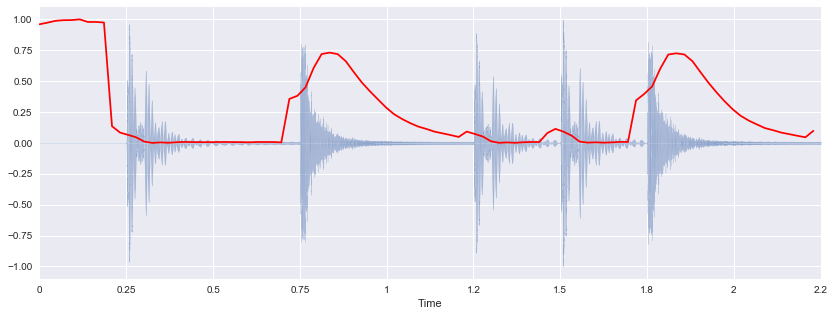Similar to the zero crossing rate, there is a spurious rise in spectral centroid at the beginning of the signal. That is because the silence at the beginning has such small amplitude that high frequency components have a chance to dominate. One hack around this is to add a small constant before computing the spectral centroid, thus shifting the centroid toward zero at quiet portions:

In :
spectral_centroids = librosa.feature.spectral_centroid(x+0.01, sr=sr)
librosa.display.waveplot(x, sr=sr, alpha=0.4)
plt.plot(t, normalize(spectral_centroids), color='r') # normalize for visualization purposes

Out:
[<matplotlib.lines.Line2D at 0x110de6a10>]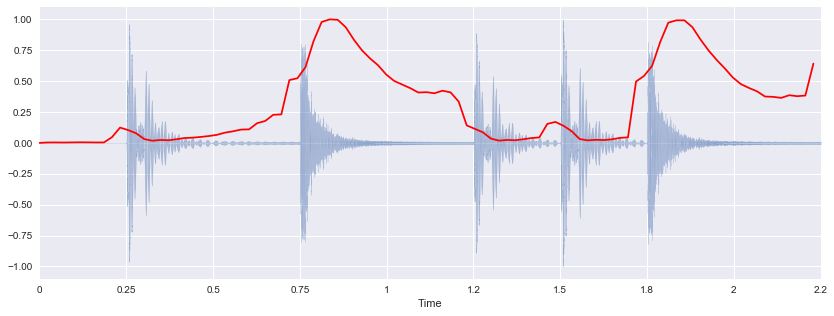## Spectral BandwidthÂ¶

librosa.feature.spectral_bandwidth computes the order-$p$ spectral bandwidth:

$$\left( \sum_k S(k) \left(f(k) - f_c \right)^p \right)^{\frac{1}{p}}$$

where $S(k)$ is the spectral magnitude at frequency bin $k$, $f(k)$ is the frequency at bin $k$, and $f_c$ is the spectral centroid. When $p = 2$, this is like a weighted standard deviation.

In :
spectral_bandwidth_2 = librosa.feature.spectral_bandwidth(x+0.01, sr=sr)
spectral_bandwidth_3 = librosa.feature.spectral_bandwidth(x+0.01, sr=sr, p=3)
spectral_bandwidth_4 = librosa.feature.spectral_bandwidth(x+0.01, sr=sr, p=4)
librosa.display.waveplot(x, sr=sr, alpha=0.4)
plt.plot(t, normalize(spectral_bandwidth_2), color='r')
plt.plot(t, normalize(spectral_bandwidth_3), color='g')
plt.plot(t, normalize(spectral_bandwidth_4), color='y')
plt.legend(('p = 2', 'p = 3', 'p = 4'))

Out:
<matplotlib.legend.Legend at 0x1110e3a50>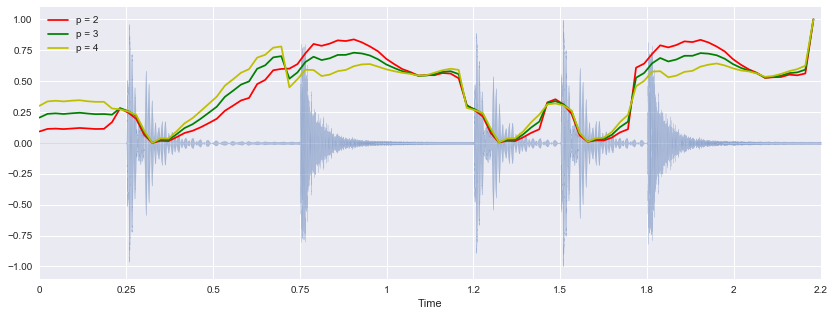## Spectral ContrastÂ¶

Spectral contrast considers the spectral peak, the spectral valley, and their difference in each frequency subband. For more information:

librosa.feature.spectral_contrast computes the spectral contrast for six subbands for each time frame:

In :
spectral_contrast = librosa.feature.spectral_contrast(x, sr=sr)
spectral_contrast.shape

Out:
(7, 97)

Display:

In :
plt.imshow(normalize(spectral_contrast, axis=1), aspect='auto', origin='lower', cmap='coolwarm')

Out:
<matplotlib.image.AxesImage at 0x113d29e50>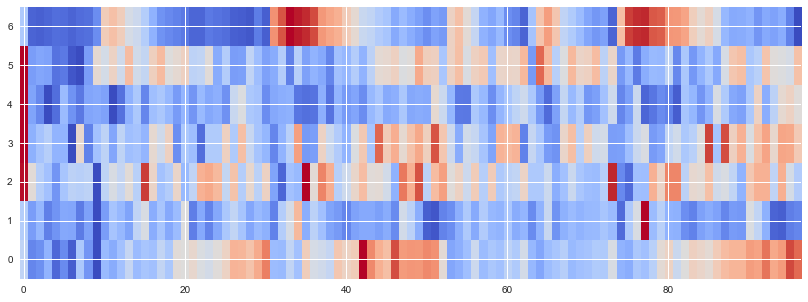## Spectral RolloffÂ¶

Spectral rolloff is the frequency below which a specified percentage of the total spectral energy, e.g. 85%, lies.

librosa.feature.spectral_rolloff computes the rolloff frequency for each frame in a signal:

In :
spectral_rolloff = librosa.feature.spectral_rolloff(x+0.01, sr=sr)
librosa.display.waveplot(x, sr=sr, alpha=0.4)
plt.plot(t, normalize(spectral_rolloff), color='r')

Out:
[<matplotlib.lines.Line2D at 0x1141df690>]